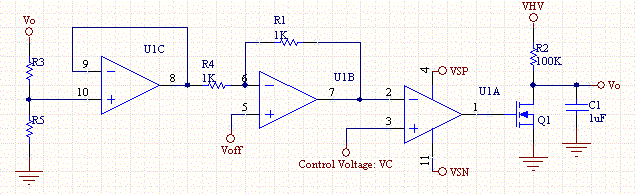# Inverse Voltage Controlled Voltage Source

This circuit allows a smaller control voltage to inversely linearly control a larger output voltage Vo. Another feature is that Vsp the supply to the op-amps can be lower than the output voltage so in theory this circuit could control a output voltage of any magnitude, since the op-amps use a different supply.

The output voltage is related to the control voltage as follows:

Vo= (R3+R5)/R3*(2*Voff-VC)

Thus, as VC gets bigger the output voltage Vo gets smaller.

This will work with single supply op-amps provided that Vsp>VCmax - Vrail, and Vsn<-- Vrail, where Vrail is the dead zone of the upper rail, this is typically .5V to 1V. This circuit will lose linearity as it attempts to drive the output voltage to zero, unless the dead zone around the rail is zero, because the control voltage approaches VS as Vo goes to zero. This non-linearity will be manifest as a rapid jump of Vo to zero, as Vc increases linearly. Some op-amps have nearly rail to rail input capability, so VS depends on the op-amp you want to use. Since as Vo goes to zero, the input to the op-amp 1C must be able to work near 0V, which can be accomplished by proper selection of a single supply op-amp, or by using a negative supply rather than ground.

The Resistors R3 and R5 scale the feedback to an appropriate range for the op-amp.

R3/(R5+R3) = (2*Voff-Vrail)/Vomax = (VCmax-Vrail)/Vomax

The offset voltage is typically half the maximum control voltage.

Voff= VCmax/2 < (VS-Vrail)/2

R3 and R5 draw current and present a small output error on the output voltage. The op-amp U1C is a unity buffer, which is needed to isolate the load from the control loop. Op-amp U1B provides gain of -1 and provides and offset voltage of 2Voff. Op-amp U1A linearizes the transistor through its large negative gain.

Capacitor C1 stops the circuit from oscillating. If the circuit oscillates, increase C1.Voltage Controlled Voltage Source Schematic

Sample values which have proven to work for the circuit are as follow:

 VHV 20V VSP 5V VSN -5V VCmax 3.3V OpAmp MC33174 R3 300K R5 39K Voff 1.65V